[TOC]

# collections

py自带的一些好用的数据结构...
https://docs.python.org/2/library/collections.html

`from collections import Counter, deque, defaultdict`

# itertools

```>>> from itertools import product, combinations
>>> a = 'ABCD'; b='EFG'
>>> for p in product(a,b …```

[TOC]

Elliot's parents speak French and English to him at home. He has heard a lot of words, but it isn't always clear to him which word comes from which language! Elliot knows one sentence that …

[TOC]

# 1. Introduction to Intractability

recall model of computation: DFA
a univeral model of computation: turing machine
→ no more powerful model of computation.
Turing machine can compute any function that can be computed by a physically harnessable process of the natural world.

bottom line: turing machine is a simple and …

[TOC]

simplex algo: top 10 algo of the 20th century (ever?).

what is linear programming:
a general problem-solving model that works for:
shortest-path, maxflow, MST, matching, assignment, ...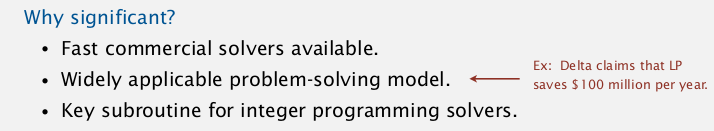# 1. Brewer-'s Problem

toy example: choose products to maximize profit.
...
feasible region: a convex polygon.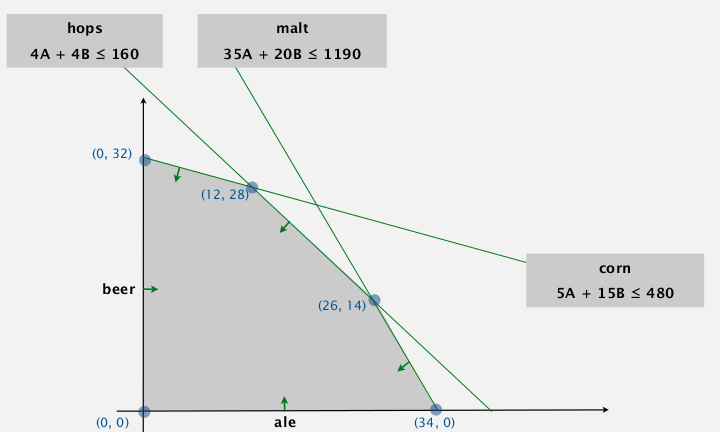⇒ optimum solution appears at an extreme point.

standard …

[TOC]

Goal: classify problems according to computational requirements.
bad new: for huge number of pbs we don't know...

# 1. Introduction to Reductions

shifing gears:

• from individual problems to problem-solving models.
• from linear/quard to polynomial/exponential pbs
• from implementation details to conceptual framwork

suppose we could (not) solve pb X …

[TOC]

# 1. Introduction to Data Compression

pb: reduce the size of a file, to save space/time for storing/transmitting.
applications: generic file compression(gzip), multimedia (mp3), communication(skype).

From binary data `B`, ⇒ generate a compressed representation `C(B)`.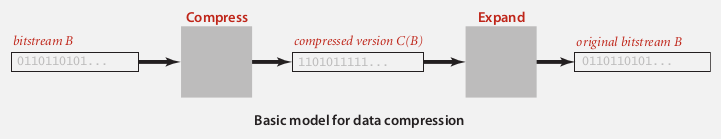lossless compression: get exactly `B` from `C(B)`
compression ratio: `|C …`

[TOC]

# 1. Regular Expressions

pb: pattern matching.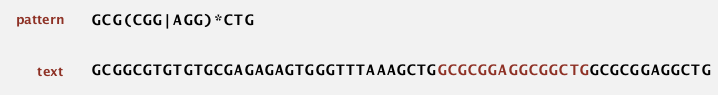### regular expression

Is a notation to specify a set of strings.
basic operations:

• concatenation
• or
• closure: "0 or more appearances of chars"
• parentheses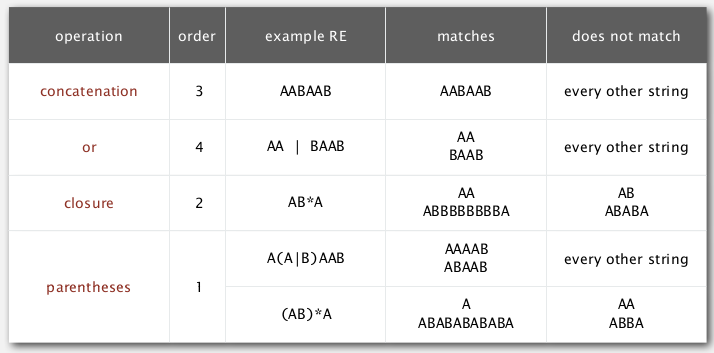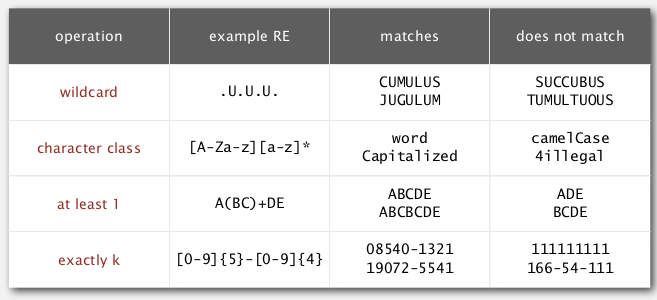ex. `[A-C]+` is equivalent to `(A|B|C)(A|B|C)*`.# 2. REs and …

[TOC]

PQ又叫"堆"(heap), 但是可能优先队列这个名字更容易记忆它的用途: pq是一种队列, 不过不是先进先出(FIFO), 而是每次出队的元素永远是优先级最高的.

# logical structure of PQ

• 除了最后一层, 所有层都排满(没有非空节点)
• 最后一层的所有非空节点都排在左边[TOC]

# 1. Introduction to substring search

"most ingenious algorithm we've seen so far"
pb. having two strings, `pattern` and `text`, len(pattern)=M << len(text)=N, try to find pattern in text.

ex. `indexOf` method of String in java.

# 2. Brute-Force Substring Search

function signature:
`public static int search(String …`

[TOC]

[Algo]

• 选择基准项(pivot element, 一般取第一个元素为pivot)
• 把数组里所有小于pivot的移动到pivot左边, 大于pivot的移动到右边 ⇒ 此时pivot已经位于最终排序时的正确位置
• 对pivot左右两个数组分别递归进行快速排序